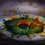# Make your solutions more attractive...

This note is a type of LaTeX guide but not about the math terms and their codes, they're seen in many notes by people.

This note is about $$\LaTeX$$ $$\color{Red}{c}\color{Orange}{o}\color{LimeGreen}{l}\color{Green}{o}\color{Blue}{r}\color{LightBlue}{s}$$.

By using the $\LaTeX$ mathematical formatting you can give $\color{#D61F06}{c}\color{#EC7300}{o}\color{limegreen}{l}\color{#20A900}{o}\color{#3D99F6}{r}\color{#95D3FE}{s}$ to your words, sentences and even math terms!!!!

For this, while wrapping the $\LaTeX$ codes in the $$.....$ \) (or the wrapping that uses " ( " in stead of " [ " ) you have to include the following code: - \text{ \color{<>respective code of color<>}{Your Text}} By this, the text you type in the second curly bracket appears in the color whose $\color{#3D99F6}{code}$ you typed in the $\textbf{first curly bracket}$. $\bullet$ For example, . " \color{Red}{Math} " will give the output $\color{#D61F06}{Math}$ , . " \color{Blue}{Mather} " will give the output $\color{#3D99F6}{Mather}$, . " \color{Green}{Mathest}" will give the output $\color{#20A900}{Mathest}$. However, if you type a sentence in the 2nd bracket, it will appear without spaces. Thus if you want a sentence to appear in colors, you have to type the following code : \text{\color{<>color code<>}{\text{Your sentence}}} $\bullet$ For example, () the code " \color{LimeGreen}{I am brilliant lover} " will appear as $\color{limegreen}{I am brilliant lover}$ () the code " \color{LimeGreen}{\text{I am brilliant lover}} " will appear as $\color{limegreen}{\text{I am brilliant lover}}$ If you want the whole sentence to be bold also along with being colored, $\color{#3D99F6}{\text{use the code}}$ " \textbf " $\color{#3D99F6}{\text{instead of}}$ " \text ". The " \color " code also works for coloring math therms, in that case you have to include whole of your math formula in the $\textbf{Second curly bracket}$. (In stead of the \text code, directly write your math term code) $\bullet$ For example, . " \color{Purple}{\dfrac{\sqrt{123}}{123\times 123}} " will give the output $\color{#69047E}{\dfrac{\sqrt{123}}{123\times 123}}$ . "\color{Green}{\binom{2^4+6^3}{7^2}} " will give the output $\color{#20A900}{\binom{2^4+6^3}{7^2}}$ Here is the list of all color codes that you might want to try. Some that are often to be used are simple, just Capitalize the first letter in the name of the color and that becomes it's code. The \color code also works on boxes when you typed the " \boxed{answer} " code in the second curly bracket. I hope this note helps you make the solutions look more attractive......... For your reference, here are some problems, while writing their solutions I have used the $\LaTeX$ $\color{#D61F06}{c}\color{#EC7300}{o}\color{limegreen}{l}\color{#20A900}{o}\color{#3D99F6}{r}\color{#95D3FE}{s}$. Quick! Before the girls come in Dancers all over !!! The Least Number!Note by Aditya Raut 7 years ago This discussion board is a place to discuss our Daily Challenges and the math and science related to those challenges. Explanations are more than just a solution — they should explain the steps and thinking strategies that you used to obtain the solution. Comments should further the discussion of math and science. When posting on Brilliant: • Use the emojis to react to an explanation, whether you're congratulating a job well done , or just really confused . • Ask specific questions about the challenge or the steps in somebody's explanation. Well-posed questions can add a lot to the discussion, but posting "I don't understand!" doesn't help anyone. • Try to contribute something new to the discussion, whether it is an extension, generalization or other idea related to the challenge. • Stay on topic — we're all here to learn more about math and science, not to hear about your favorite get-rich-quick scheme or current world events. MarkdownAppears as *italics* or _italics_ italics **bold** or __bold__ bold - bulleted- list • bulleted • list 1. numbered2. list 1. numbered 2. list Note: you must add a full line of space before and after lists for them to show up correctly paragraph 1paragraph 2 paragraph 1 paragraph 2 [example link](https://brilliant.org)example link > This is a quote This is a quote  # I indented these lines # 4 spaces, and now they show # up as a code block. print "hello world" # I indented these lines # 4 spaces, and now they show # up as a code block. print "hello world" MathAppears as Remember to wrap math in $$ ... $$ or \[ ... $ to ensure proper formatting.
2 \times 3 $2 \times 3$
2^{34} $2^{34}$
a_{i-1} $a_{i-1}$
\frac{2}{3} $\frac{2}{3}$
\sqrt{2} $\sqrt{2}$
\sum_{i=1}^3 $\sum_{i=1}^3$
\sin \theta $\sin \theta$
\boxed{123} $\boxed{123}$

Sort by:

$\color{lightpink}{\text{Oooh, pink.}}$

- 6 years, 11 months ago

$\color{magenta} {\displaystyle\int_{0}^{\infty}}$$\color{#D61F06}{sin} ^{2}\color{#E81990}{x}$.$\color{#20A900}{dx}$

$\color{#0C6AC7}{Ooooh}.. \color{limegreen}{multicolor}$

- 6 years, 7 months ago

Thanks for sharing.

- 7 years ago

@Calvin Lin sir, the following problems are still not given rating but i feel they're not so bad!

143 is special

GCD !

- 7 years ago

... It took me so long to get it correct... I was wondering what was wrong when I realized I spelled color as colour

- 6 years, 10 months ago

Difference of American and British english right ? @Tan Wee Kean

- 6 years, 10 months ago

Yes! My school is quite strict about it. :(

- 6 years, 10 months ago

How do u add the line after each section?

- 6 years, 9 months ago

Just type the underscore thing 3 times. (the horizontal line in aditya _ raut)

So typing '_ _ _' (without spaces) gives the white line as follows.

Hope that helps @Aneesh Kundu

- 6 years, 9 months ago

Thnx

- 6 years, 9 months ago

Why did you used \color{#3D99F6}{\text{\dfrac}} instead of \color{#D61F06}{\text{\frac}}

- 6 years, 8 months ago

That is to ensure clearer display, because \dfrac gives a bigger display of the fraction that \frac.

Try it and you'll notice the difference :)

- 6 years, 8 months ago

Using \frac: $\frac{1}{2}$

Using \dfrac: $\dfrac{1}{2}$

- 6 years, 8 months ago

Cool! Thanks!

- 6 years, 8 months ago

$\color{#20A900}{15 \sin x}$

- 3 years, 8 months ago

$\color{#3D99F6}{\text{Wow, it`s so cool!}}$

- 6 years, 9 months ago

Dude you got to wrap it with the formatting

\ ( {your codes} \ )

Type the above without spaces and then it will appear.

- 6 years, 9 months ago

thank you , iam pretty much rubbish at latex.

- 6 years, 9 months ago

Now I know LaTeX pretty well

- 5 years, 7 months ago